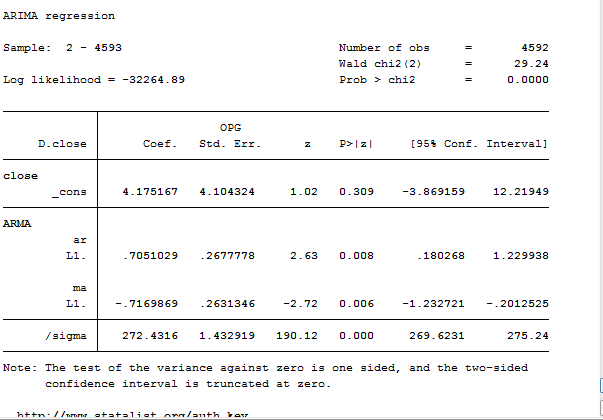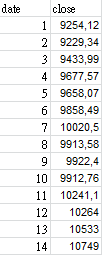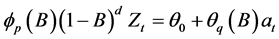# how to input real data into arima model?

Member Posts: 5Contributor I
edited December 2018 in Help

hello

I am a beginner of arima, and wanna ask some questionafter I found the arima (1,0,1) coefficients, how can I actually use them into real data like below in excel:I've read many of the articles, they only provide the original algebra equation,something like this, which I wanna know is how to use in pratical.

If I am gonna predict the price of date 15, which column should multiply which coefficient to make the result come out?

Thanks very much

Tagged:

• Posts: 263Unicorn
Solution Accepted

Y(t) = 4.17 + 0.70* Y(t-1) + e(t) - 0.71* e(t-1)

This means you need a series for Y(t) and another one for e(t). It looks to me the program you are using is Stata (and some in this forum will ask : Why didn't you ask the question in a Stata forum).

You can get the series for the residual ( e(t) above) using:

predict r, resid

Now plug in the values and you'll get your forecast.

Of course, you could directly get the forecast in Rapidminer or in Stata with the appropriate command.

predict r, resid

• 12Administrator, Moderator, Employee, RapidMiner Certified Analyst, Community Manager, Member, University Professor, PM Moderator Posts: 2,876Community Manager

hello @marielong - welcome to the User Community.  Did you do a search for "ARIMA" in the "Search the community..." bar above?  There is a thread on exactly this question that has been taking place for the past week (http://community.rapidminer.com/t5/Getting-Started-Forum/How-future-predictions-can-be-made-with-a-Time-Series-model-in/m-p/42249#M1002) as well as a KB article.

Hope that helps.

Scott

• Member Posts: 5Contributor I

Hello earnijo, thanks for your kindnessIt came out like this

date15 value= 4.17 + 0.70*10749 - 0.71* 217,555 = 7427,35

is it right?

so if the predicted price is so far from the real date 15 value(10662,8), can it be said that my model (1 0 1) is wrong?

• Member Posts: 5Contributor I

Hello scott, thanks for help

• Member Posts: 263Unicorn

Marielong:

Your computation is correct. But I would not use the prediction of one observation as a sign of failure (or success if it was really close). You can get lucky. The difference between your prediction and the actual value (if the model is true) is e(t). By definition e(t) cannot be forecast.

If you had more observations (many more than one), you could compare the performance of different models using a metric like the out-of-sample RMSE.

• Member Posts: 5Contributor I

Dear earmijo,

thanks for you reply again, it was helpfulBut I still have some questions about the model since I am only a beginner, still seeking for your help.

e(t) comes from newer actual value - older actual value, it should be correct

1.if e(t) = my "r" column, why you've mentioned e(t)=actual value - predicted value, but not =newer actual value - older actual value like in "r' column?

2.I'm a bit confused eg:

date   actual value   predicted value

1             10                   12

2             21                   22

3             30                   31

e(t) case 1: actual newer - older = 30 - 21 = 9

case 2: actual - predicted = 30 - 31 = -1

it seems a quite big difference, there's why I can't understand

which case should I use?

3.if case 2, to make model like (1 1 1), it has to find a series of e(t) first to compute the equation, but how to figure out e(t) as the predicted value is an unknown?

4.if the model is (1 1 0), the AR coefficient is negative, the result comes out will be negative too, so that the model is wrong?

5.if the model is (0 1 1), means MR coefficient x e(t-1), but the residual must be much smaller than the actual value, so it comes out the result predicted value will be very small, the model goes to false too?

Sorry that I have many questions.

• Member Posts: 263Unicorn

e(t) comes from newer actual value - older actual value, it should be correct

1.if e(t) = my "r" column, why you've mentioned e(t)=actual value - predicted value, but not =newer actual value - older actual value like in "r' column?

The correct formula is:

e(t) = y(t) - pred y(t)

pred y(t) =  4.17 + 0.70* y(t-1) - 0.71* e(t-1)

If your 'r' column was computed as the difference of the series, then you were not computing e(t) but something else.

Suposse you run the following commands:

arima close, arima(1,0,1)

predict r, resid

predict yhat, y

generate e = close - yhat

list close p r e

Then e and r are identical.

2.I'm a bit confused eg:

date   actual value   predicted value

1             10                   12

2             21                   22

3             30                   31

e(t) case 1: actual newer - older = 30 - 21 = 9

case 2: actual - predicted = 30 - 31 = -1

it seems a quite big difference, there's why I can't understand

which case should I use?

Use case 2

3.if case 2, to make model like (1 1 1), it has to find a series of e(t) first to compute the equation, but how to figure out e(t) as the predicted value is an unknown?

No. You only need the y(t) series. The e(t) is obtained after estimation (using the definition above).

4.if the model is (1 1 0), the AR coefficient is negative, the result comes out will be negative too, so that the model is wrong?

arima(1,1,1) is an arima(1,0,1) for the difference of the series ( delta y(t) = y(t) - y(t-1) ). So it is no problem if you get a negative forecast.

5.if the model is (0 1 1), means MR coefficient x e(t-1), but the residual must be much smaller than the actual value, so it comes out the result predicted value will be very small, the model goes to false too?

Same comment as above. arima(0,1,1) is an arima (0,0,1) for the difference of the series. You are forecasting the difference in the series. That one probably is smaller than the series itself.

I would look into a library written by Rob Hyndman to automate the selection of the best model using AIC, BIC or other information criteria. But it is written for R.

Sorry that I have many questions.

• Member Posts: 5Contributor I

Thank you very much earmijo